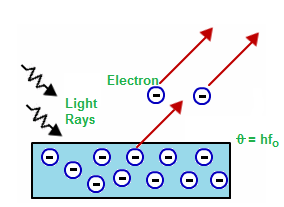# Einstein’s Photoelectric Equation and Laws of Photoelectric Equation

Einstein’s photoelectric equation: Einstein gave an explanation for photoelectric emission based on the Planck’s quantum theory of light.Emission of electron from a metallic surface

When light of certain frequency (greater than threshold frequency) incidents on a metallic surface the energy on incident photon (hf) is:

• Partly used to eject the electron from surface.
• Partly remains as kinetic energy of ejected electrons.

i.e. Energy of photon = Work Function + Kinetic Energy of Electrons

or, $${hf = \phi + \text{Kinetic Energy} }$$
$${hf = hf_{\circ} + \text{Kinetic Energy}}$$

$$\frac{ch}{\lambda } = \frac{ch}{\lambda_\circ } = \frac{1}{2}mv^{2}$$

Where, h = Planck’s constant = 6.63×10-34 Js

Laws of photoelectric emission:

• There exists a minimum frequency of incident light below which photoelectric emission is no possible. The frequency is called threshold frequency.
• A part of energy of incident electrons is used to eject electrons from a surface and remaining as kinetic energy of the ejected electrons.
• Increase in intensity of light increases the number of photoelectron.
• Increase in frequency of light increases the kinetic energy of electrons.

Do you like this article ? If yes then like otherwise dislike : 32

#### 2 Responses to “Einstein’s Photoelectric Equation and Laws of Photoelectric Equation”

1.Talim

What are eddy current?

•Sourabh

Talim ,eddy current is the produce, when a metal plate is suspended in strong magnetic field, when its rotate current is produce in it by the force exert by magnetic field habe all possible direction.@